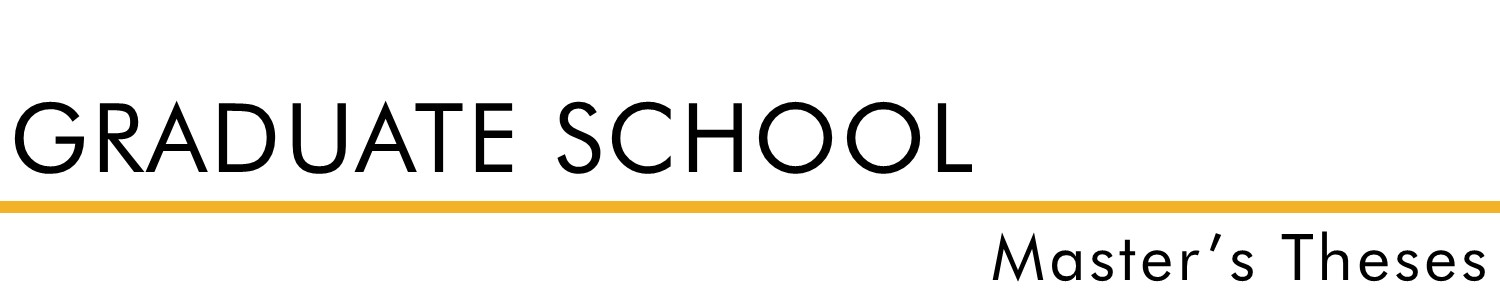#### Title

Orthogonal Functions

#### Document Type

Thesis - campus only access

Spring 1956

#### Degree Name

Master of Science (MS)

#### Department

Math

Dr. Emmet C. Stopher

#### Abstract

It was the purpose of this thesis (1) to investigate certain known orthogonal functions; (2) to exhibit elements of similarity among them; (3) to show that they arise as the solutions of differential equations in a similar way; (4) to establish that the differential equations which yield them are similar and are special cases of yet another more general differential equation; (5) to verify that the Sturm-Liouville theory can be applied to each of these differential equations; and (6) to demonstrate how each of these orthogonal functions is used to give a series representation of a given function. By direct computation it was shown that the orthogonal functions studied arose from their respective differential equations in a similar way. These differential equations were then shown to be special cases of a more general differential equation, thus showing another similarity among the orthogonal functions. The Sturm-Liouville theory was used to find certain information in order to establish the orthogonality of the functions that were a solution of the various differential equations. Finally, by direct methods each of the orthogonal functions was utilized in forming a series representation of two arbitrary functions and these series representations were put in graphic form to show yet another similarity of the orthogonal functions that were studied.

For questions contact ScholarsRepository@fhsu.edu

#### Rights

© 1956 Arnold L. Janousek

COinS